Standard Units (SI Units)

The International System of Units (abbreviated SI) is the metric system used in science, industry, and medicine.

Learning Objectives

Recognize SI units and their importance for measurment

Key Takeaways

Key Points

• Every field of science involves taking measurements, understanding them, and communicating them to others. In other words, we all have to speak the same basic language.
• The SI system, also called the metric system, is used around the world.
• There are seven basic units in the SI system: the meter (m), the kilogram (kg), the second (s), the kelvin (K), the ampere (A), the mole (mol), and the candela (cd).

Key Terms

• SI system: A series of units that is accepted and used throughout the scientific world.

The Need for a Common Language

Every field of science involves taking measurements, understanding them, and communicating them to others. In other words, we all have to speak the same basic language. Whether you are a chemist, a physicist, a biologist, an engineer, or even a medical doctor, you need a consistent way of communicating size, mass, shape, temperature, time, amount, energy, power, and speed.

Consider the screen on which you’re reading this text right now. It might be an LCD screen, which is made up of liquid crystals. The chemist developing a specific formulation for a liquid crystal has to meaningfully communicate information to an engineer so that the engineer knows how to manufacture it. The engineer, in turn has to be able to communicate with other engineers, physicists, and chemists to design the circuit boards, display screens, and electronic interfaces of the rest of the computer. If these people don’t all speak the same language, the enterprise will never get off the ground.

The International System of Units (abbreviated SI, from the French Système international d’unités) is the metric system used in science, industry, and medicine. Depending on your age and geographic location, you might be very familiar with the “imperial” system, which includes units such as gallons, feet, miles, and pounds. The imperial system is used for “everyday” measurements in a few places, such as the United States. But in most of the world (including Europe) and in all scientific circles, the SI system is in common use.

Scientific Units the SI and Metric Units: Mr. Causey teaches scientific units of the SI system, the metric system, and the CGS system. Mr. Causey also shares the major prefixes and their meanings. Science measurements are based on the metric system, so it is important that you know the metric base units and prefixes.

Units of the SI System

There are seven base units in the SI system:

• the kilogram (kg), for mass
• the second (s), for time
• the kelvin (K), for temperature
• the ampere (A), for electric current
• the mole (mol), for the amount of a substance
• the candela (cd), for luminous intensity
• the meter (m), for distance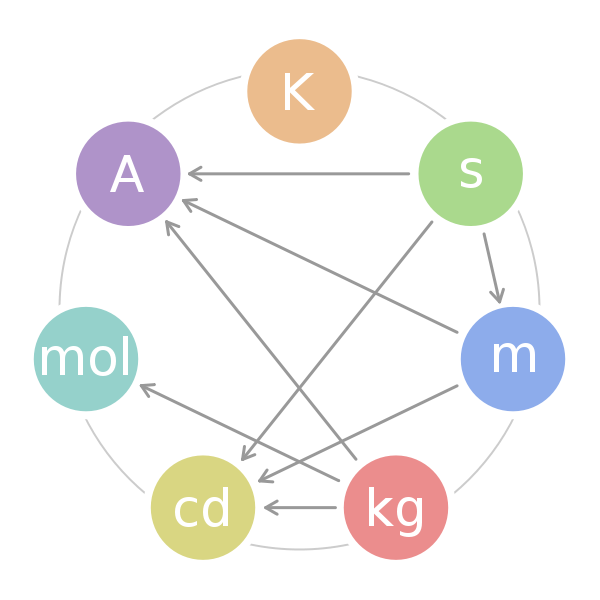The Seven SI Units: This figure displays the fundamental SI units and the combinations that lead to more complex units of measurement.

It should be apparent that the move into modern times has greatly refined the conditions of measurement for each basic unit in the SI system, making the measurement of, for example, the luminous intensity of a light source a standard measurement in every laboratory in the world. A light source made to produce 20 cd will be the same regardless of whether it is made in the United States, in the UK, or anywhere else. The use of the SI system provides all scientists and engineers with a common language of measurement.

History of the SI System

The SI units of measurement have an interesting history. Over time they have been refined for clarity and simplicity.

• The meter (m), or metre, was originally defined as 1/10,000,000 of the distance from the Earth’s equator to the North Pole measured on the circumference through Paris. In modern terms, it is defined as the distance traveled by light in a vacuum over a time interval of 1/299,792,458 of a second.
• The kilogram (kg) was originally defined as the mass of a liter (i.e., of one thousandth of a cubic meter). It is currently defined as the mass of a platinum-iridium kilogram sample maintained by the Bureau International des Poids et Mesures in Sevres, France.
• The second (s) was originally based on a “standard day” of 24 hours, with each hour divided in 60 minutes and each minute divided in 60 seconds. However, we now know that a complete rotation of the Earth actually takes 23 hours, 56 minutes, and 4.1 seconds. Therefore, a second is now defined as the duration of 9,192,631,770 periods of the radiation corresponding to the transition between the two hyperfine levels of the ground state of the cesium-133 atom.
• The ampere (A) is a measure of the amount of electric charge passing a point in an electric circuit per unit time. 6.241×1018 electrons, or one coulomb, per second constitutes one ampere.
• The kelvin (K) is the unit of the thermodynamic temperature scale. This scale starts at 0 K. The incremental size of the kelvin is the same as that of the degree on the Celsius (also called centigrade) scale. The kelvin is the fraction 1/273.16 of the thermodynamic temperature of the triple point of water (exactly 0.01 °C, or 32.018 °F).
• The mole (mol) is a number that relates molecular or atomic mass to a constant number of particles. It is defined as the amount of a substance that contains as many elementary entities as there are atoms in 0.012 kg of carbon-12.
• The candela (cd) was so named to refer to “candlepower” back in the days when candles were the most common source of illumination (because so many people used candles, their properties were standardized). Now, with the prevalence of incandescent and fluorescent light sources, the candela is defined as the luminous intensity in a given direction of a source that emits monochromatic radiation of frequency $540 \cdot 10^{12}$ Hertz and that has a radiant intensity in that direction of 1/683 watts per steradian.

SI Unit Prefixes

The basic SI units can be expressed as fractions and multiples of basic units by using a set of simple prefixes.

Learning Objectives

Convert between SI units

Key Takeaways

Key Points

• The set of prefixes is simple and easy to use.
• Prefixes cannot be combined.
• The set of prefixes is universal.

Key Terms

• prefix: One or more letters or syllables added to the beginning of a word to modify its meaning; for example, kilo can be added to gram to create kilogram
• fraction: a part of a whole, especially a comparatively small part

SI Unit Prefixes

Now that we know about the SI system and what it provides to the scientist and engineer, we can examine some aspects of actual measurement. The SI system utilizes a standard system of prefixes to the basic units that allow them to be more relevant to and descriptive of relative magnitude.

For example, when reading about chemical kinetics, you may encounter the terms “ms” or “ns,” meaning “millisecond” and “nanosecond” respectively. Once you’ve become accustomed to the practice of using the prefixes, you will immediately know that the millisecond is 1/1000 of one second and is 1 million times larger than a nanosecond, which is 1/1000000000 of one second, or 10-9 seconds.

Briefly review the basic SI units before you study the prefixes.

Unit name Unit symbol Quantity name Quantity symbol Dimension symbol
meter m length l, x, r L
kilogram kg mass m M
second s time t T
ampere A electric current I I
kelvin K thermodynamic temperature T Θ
candela cd luminous intensity Iv J
mole mol amount of substance n N

There are 20 accepted prefixes. A prefix may be used to identify multiples of the original unit or fractions of the original unit. For example, kilo- denotes a multiple of a thousand, so there are one thousand meters in a kilometer. Milli- denotes a thousandth; therefore, there are one thousand millimeters in a meter.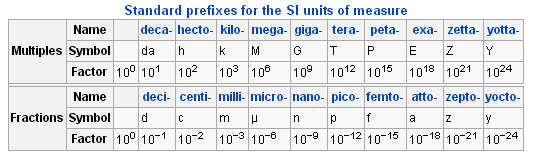Prefixes for SI units: The prefixes redefine the measurement as either a multiple or a fraction of the basic unit.

Keep in mind that prefixes should never be combined. Thus a millionth of a meter is a micrometer, not a millimillimeter, and a millionth of a kilogram is a milligram, not a microkilogram.

In older usage, a micron (a measurement often encountered in physics and engineering) is the same as a micrometer, 10-6 meters. Another older form of usage, the millimicron, is one thousandth of a micrometer, or 1 thousandth of 10-6 meters, or 10-9 meter, now called a nanometer. While these older terms are not in common usage, they are often encountered in older publications, and knowing their modern equivalents is an advantage.

Volume and Density

Density and volume are two common measurements in chemistry.

Learning Objectives

Describe the relationship between density and volume

Key Takeaways

Key Points

• The volume of a substance is related to the quantity of the substance present at a defined temperature and pressure.
• The volume of a substance can be measured in volumetric glassware, such as the volumetric flask and the graduated cylinder.
• Density indicates how much of a substance occupies a specific volume at a defined temperature and pressure. The density of a substance can be used to define the substance.
• Water is unusual because when water freezes, its solid form (ice) is less dense than liquid water, and thus floats on top of liquid water.

Key Terms

• volume: A unit of three-dimensional measure of space that comprises a length, a width, and a height. It is measured in units of cubic centimeters in metric.
• density: A measure of the amount of matter contained in a given volume.

Volume and Density

The properties of a material may be described in many ways. Any amount of any substance will have a volume. If you have two containers of water that are different sizes, they each hold a different amount, or volume, of water. The unit for volume is a unit derived from the SI unit of length and is not a fundamental SI measurement.

If two water samples have different volumes, they still share a common measurement: the density. Density is another measurement derived from SI basic units. The density of a material is defined as its mass per unit volume. In this example, each volume of water is different and therefore has a specific and unique mass. The mass of water is expressed in grams (g) or kilograms (kg), and the volume is measured in liters (L), cubic centimeters (cm3), or milliliters (mL). Density is calculated by the dividing the mass by the volume, so that density is measured as units of mass/volume, often g/mL. If both water samples are at the same temperature, their densities should be identical, regardless of the samples’ volume.

Measurement Tools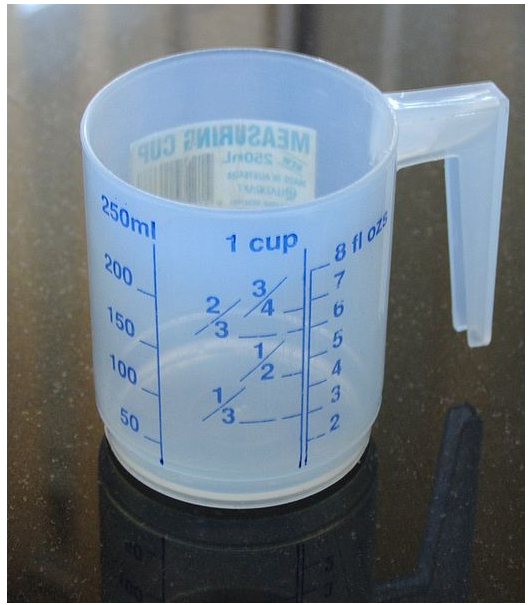The measuring cup: The measuring cup is a common household utensil used for measuring the volumes of liquids.

If you have ever cooked in a kitchen, you have probably seen some sort of measuring cup, which allows the user to measure liquid volumes with reasonable accuracy. The measuring cup expresses liquid volume in the standard SI units of liters and milliliters. Most American measuring cups also measure liquid in the older system of cups and ounces.

Volumetric Glassware

Scientists who work in a laboratory must be familiar with typical laboratory glassware, often called volumetric glassware. These may include beakers, a volumetric flask, an Erlenmeyer flask, and a graduated cylinder. Each of these containers is used in a laboratory setting to measure liquid volumes for different purposes.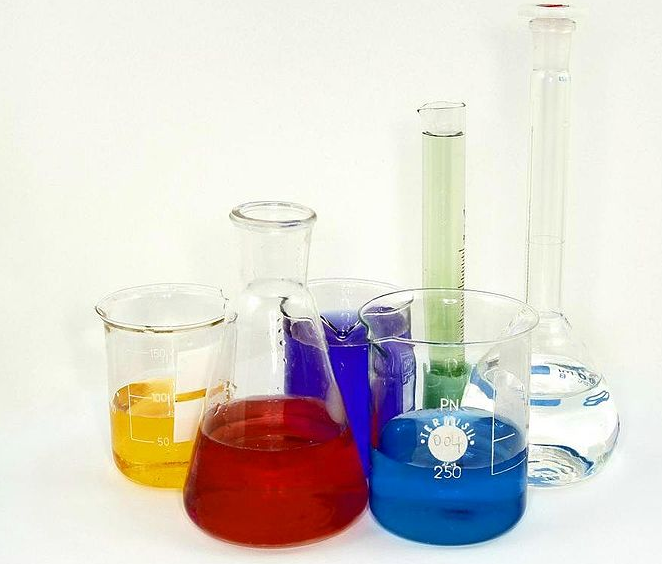Laboratory volumetric glassware: Glassware, such as these beakers, is commonly used in a laboratory setting to conveniently measure and separate different volumes of liquids.

Density of Water

Different substances have different densities, so density is often used as a method to identify a material. Comparing the densities of two materials can also predict how substances will interact. Water is used as the common standard to substances, and it has a density of 1000 kg/m3 at Standard Temperature and Pressure (called STP).

Using Water as a Density Comparison

When an object is placed in water, the object’s relative density determines whether it floats or sinks. If the object has a lower density than water, it will float to the top of the water. An object with a higher density will sink. For example, cork has a density of 240 kg/m3, so it will float. Air has a density of approximately 1.2 kg/m3, so it rises immediately to the top of a water column. The metals sodium (970 kg/m3) and potassium (860 kg/m3) will both float on water, while lead (11,340 kg/m3) will sink.

Density: A Story of Archimedes and the Gold Crown: Is the crown made of pure gold? An ancient Greek king needs to know if he’s been cheated by a goldsmith. He summons Archimedes, who decides to use density to determine the metal. But how can he determine the volume of the crown?

Liquids tend to form layers when added to water. The sugar alcohol glycerol (1,261 kg/m3) will sink into the water and form a separate layer until it is thoroughly mixed (glycerol is soluble in water). Vegetable oil (approx. 900 kg/m3) will float on water, and no matter how vigorously mixed, will always return as a layer on the water surface (oil is not soluble in water).

The Variable Density of Water

Water itself is a complicated and unique molecule. Even if the pressure is consistent, water’s density will change based on the temperature. Recall that the three basic forms of matter are solid, liquid and gas (ignore plasma for the time being). As a rule of thumb, almost all materials are more dense in their solid or crystalline form than in their liquid form; place the solid form of almost any material on the surface of its liquid form, and it will sink. Water, on the other hand, does something very special: ice (the solid form of water) floats on liquid water.

Look carefully at the relationship between water’s temperature and its density. Beginning at 100 °C, the density of water steadily increases, as far as 4 °C. At that point, the density trend reverses. At 0 °C, water freezes to ice and floats.

This table lists the densities of water at different temperatures and constant pressure.

The density of water at constant pressure
Temp (ºC) Density (kg/m3)
100 958.4
80 971.8
60 983.2
40 992.2
30 995.6502
25 997.0479
22 997.7735
20 998.2071
15 999.1026
10 999.7026
4 999.9720
0 999.8395
−10 998.117
−20 993.547
−30 983.854
The values below 0ºC refer to super cooled water

The implications of this simple fact are enormous: when a lake freezes, ice crusts at the surface and insulates the liquid below from freezing, while at the same time allowing the colder water (with a temp of approx. 4 °C and a high density) to sink to the bottom. If ice did not float, it would sink to the bottom, allowing more ice to form and sink, until the lake froze solid! Scuba divers and swimmers often encounter these water temperature gradients, and they might even encounter a water layer at the very bottom of a lake with a temperature of approximately 4 °C. That’s just about as cold as the lake will get at the bottom; as soon as the water gets colder, the liquid water becomes less dense and rises.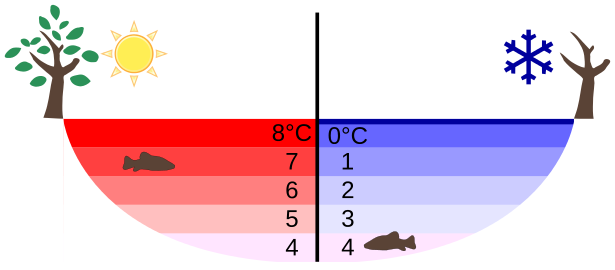Layers of water in a winter lake: During the winter months of seasonal climates, the warmest water in most lakes and rivers is only 4°C. This 4°C water has the highest density and sinks to the bottom of the lake. As the water becomes colder (<4°C), it becomes less dense and rises to form ice on the surface of the lake. As a result, liquid water always exists in lakes and rivers during the winter months. This unique property of water enables animals and plants to survive under the frozen lake or winter, ensuring that all freshwater life does not go extinct each winter.

Temperature

The ability to measure temperature accurately was a major scientific advancement, putting absolute numbers on an observable phenomenon.

Learning Objectives

State the key developments in the history of temperature measurement

Key Takeaways

Key Points

• The measurement of temperature is accurate and reproducible.
• The measurement of temperature should be against accepted standards.
• Temperature can be calibrated to several scales including Celsius, Fahrenheit, and kelvin.
• Converting between different temperature scales is easy using the conversion equations.
• Kinetic energy arises from the motion of atoms and molecules. It is postulated that at absolute 0, there is no motion and therefore, no kinetic energy.

Key Terms

• temperature: A measure of cold or heat, often measurable with a thermometer.
• kelvin: A unit of measurement for temperature. It is one of the seven base units in the International System of Units (SI).
• Fahrenheit: An unit of measurement for temperature used most commonly in the United States.
• Celsius: A scale and unit of measurement for temperature where 0 °C is the freezing point of water. Also known as centigrade.
• Celsius: A scale and unit of measurement for temperature where 0 °C is the freezing point of water. Also known as centigrade.

How hot was it last summer? Will it be cold enough for skiing next week? Each of these questions forces quantification to a routine experience. Whether we’re talking about the weather, cooking food, or conducting a scientific experiment, we need to know how hot or how cold something is. To know this, one must be able to put some sort of accurate number on the concept. Although there is an entire field of study devoted to measuring temperature (thermometry), the focus of this section is on the fundamental measurements of temperature.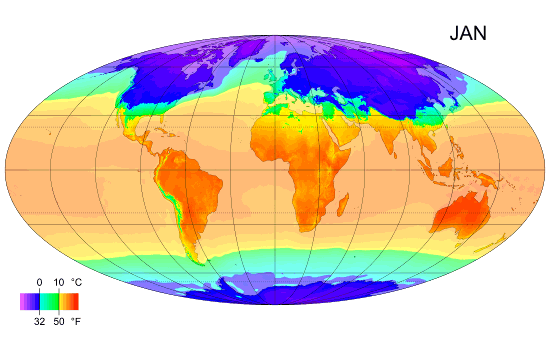Monthly mean temperature: Temperature enables us to accurately measure and compare climates in different parts of the world.

The History of Measuring Temperature

To people in the 21st century, measuring temperature is a quick and easy thing to do. Thousands of years ago, however, things were different. Temperature-related phenomena were always being observed. Snow fell and collected in cold weather, and melted into liquid water when the air warmed in spring. Liquid water fell as rain when the air was warm. Ice melted when placed near a source of heat, and water completely boiled out of a pot on a hot stove. However, these are all qualitative observations. They do not generate a number: they do not tell us that water freezes at 0 °C, or that it boils at 100 °C. All we learn from observation is that heat and cold do something to water, or that water behaves differently when it is heated or cooled.

In the 16th and 17th centuries, scientists refined the observations and experiments of the Byzantines and Greeks to produce rudimentary devices relating the amount of “hotness” or “coldness” in the air. The devices they built were called thermoscopes. These basic measuring tools utilized the expansion and contraction of air and water when heated and cooled.

The concept was remarkable, but thermoscopes did not have a numeric scale. The thermoscope could not answer the question, “How hot is it today?” with a number, but it could give a relative measurement. The thermoscope was often a simple tube of gas over liquid. Thermoscopes also served as barometers (which measure pressure ). That made it difficult to use them as thermometers, but they reacted to both pressure and temperature. Even when early thermometers did have a numeric scale, the scales were not standardized.

The dawn of the 18th century saw great change in thermometers, thanks to the work of Isaac Newton, Anders Celsius, and Daniel Fahrenheit.

• Isaac Newton proposed a thermometer with a scale of 12 degrees between the freezing and boiling points of water.
• Fahrenheit was working with tubes filled with mercury, which has a very high coefficient of thermal expansion. This, combined with the quality and accuracy of Fahrenheit’s work, led to much greater sensitivity, and his thermometer was standardized against a brine solution and universally adopted, with the Fahrenheit scale being named in his honor.
• Anders Celsius proposed a 100 degree scale for the difference between freezing and boiling of water, and after a few minor adjustments, the Celsius, or centigrade, system was also widely adopted.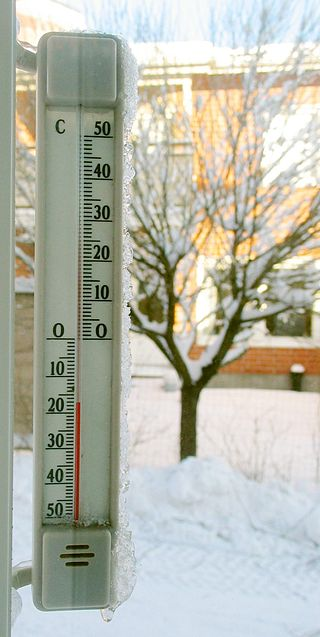Thermometer calibrated with the Celsius scal: Celsius is a scale and unit of measurement for temperature where 0 °C is the freezing point of water. Our ability to accurately measure temperature enables us to measure the weather, cook food accurately, or conduct a scientific experiment.

Further advances led to faster-acting thermometers, which were useful in medicine and chemistry. Early thermometers did not record or hold the temperature they were measuring: if you removed the thermometer from the substance being measured, its reading would change. Scientists invented new thermometers that would maintain their reading, at least for a limited period of time, to reduce measurement errors and make it easier to record the temperature. Dial thermometers using bimetallic strips were also developed. The bimetallic strips are made from two dissimilar metals bonded together, with each metal having a different coefficient of thermal expansion. Upon heating or cooling, the two metals expand or contract at different rates, causing a bending or curvature to appear in the strip. This bending is useful as a transducer for the temperature reading; it can control a thermostatted circuit or drive a simple dial thermometer.

Absolute Zero

Through the development of temperature measurement, however, one question remained unanswered: “How cold can it really get? How cold is absolute 0?”

The trivial answer is “0 degrees,” but what exactly does that mean? Temperature itself is the measurement of the average kinetic energy of a substance. The kinetic energy arises from the motion of atoms and molecules, and it is postulated that at absolute 0, there is no motion and therefore, no kinetic energy. Therefore, the temperature must be “absolute 0.”

The question remains: how much colder is absolute 0 than 0 °C?

In 1848, Lord Kelvin (William Thomson) wrote a paper entitled “On An Absolute Thermometric Scale” about the need to seek out a thermodynamic zero temperature. Using the Celsius system for its measurement of degrees, Lord Kelvin calculated the ultimate cold temperature to be -273 °C. Today that is referred to as 0 K on the Kelvin thermodynamic temperature scale. Modern methods have refined the measurement to -273.16 °C.

Types of Temperature Scales

Temperature can be measured and represented in many different ways. The fundamental requirements of the practice involve accuracy, a standard, linearity, and reproducibility. The SI unit, chosen for its simplicity and relationship to thermodynamics, is the kelvin, named in honor of Lord Kelvin. While incrementally equal to the Celsius scale, the temperature in kelvins is a true representation of the kinetic energy in a thermodynamic sense. Chemistry and physics require many calculations involving temperature. Those calculations are always made in kelvins.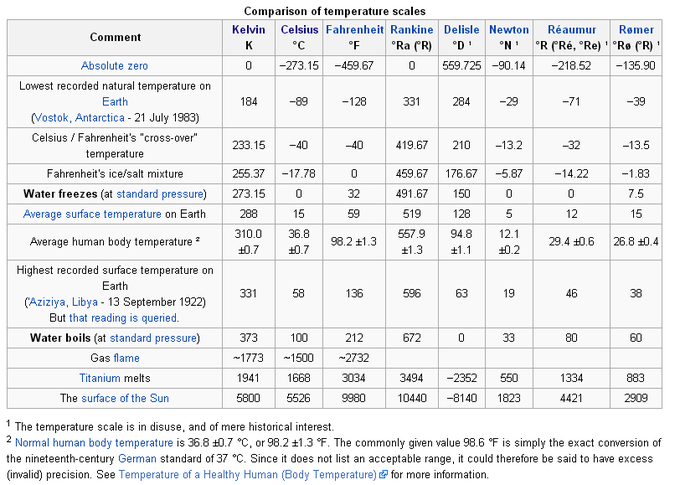Comparison of temperature scales: Temperatures of some common events and substances in different units.

A comparison of temperature scales table illustrates a variety of temperature scales, some of which are no longer used. It is interesting to see the temperatures of commonly occurring events over these scales, and to imagine the great hurdles that were overcome in developing modern thermometry.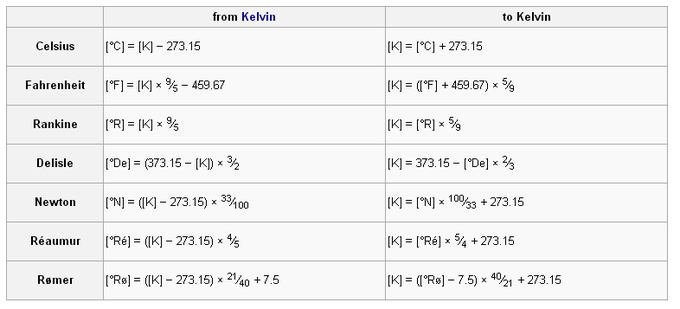Conversion to and from kelvin: Use the equations in this table to calculate temperatures using the kelvin measurement system.

Although in most cases scientists are equipped with some sort of electronic calculator, there might be times when a conversion from one scale to another is required. Conversion tables can be used to convert a measurement to any scale from any other temperature scale, such as kelvin or Celsius.Conversion to and from degrees Celsius: Use the equations in this table to convert temperatures to the Celsius measurement system.

1. Despite the prefix "kilo-," the kilogram is the base unit of mass. The kilogram, not the gram, is used in definitions of derived units. Nonetheless, units of mass are named as if the gram were the base unit.